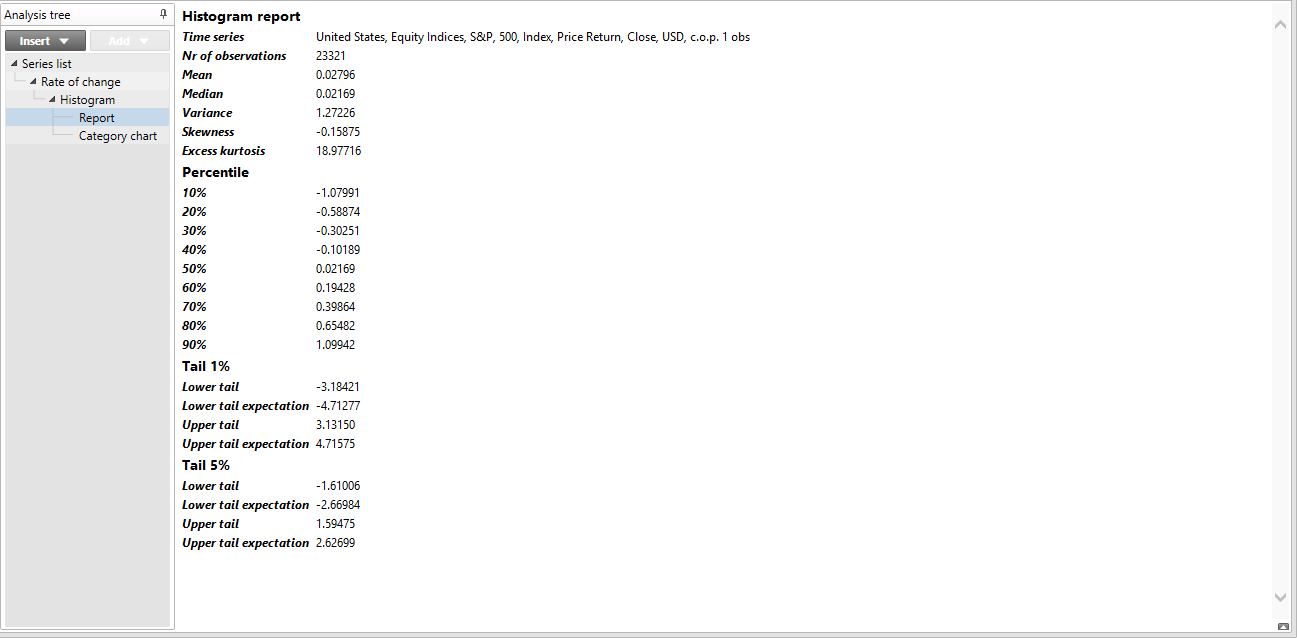# Overview

With the Histogram analysis, you can view the distribution of values of one or more series. It’s typically presented as a report containing the main distribution statistics and as a category chart displaying the distribution among bins. Use it if you want to know whether values are within normal limits or if they’re skewed in one direction.

# Settings## Density vs. Cumulative distribution

This setting determines if the density or the cumulative distribution should be estimated.

• Density: measures the items that fall into each individual bin.
• Cumulative distribution: measures the items that fall into each bin plus all previous bins.

## Relative vs. Count

This setting determines the unit of the histogram.

• Relative: the unit is the proportion of items per bin.
• Count: the unit is the absolute number of items per bin.

## Method

### Methods available when density is selected

• Uniform kernel: measures the number of items that falls exactly into each bin.
• Normal kernel: uses a smooth windowing function based on the standard normal density function when calculating the value for each bin.

### Methods available when cumulative distribution is selected

• Uniform: measures the number of items until the end of each bin.
• Empirical: is calculated not by dividing the range into bins, but by using the actual points of observations.

## Start & End

Here, you can specify a data sample range. If left blank, the whole series is used.

## Auto bin

If checked, the application will automatically calculate a bin size as described in the theory section below.

## Width

Here, you can specify the bin size if the automatic bin size is turned off.

## Normal

This contains options for outputting a normal distribution series.

• None does not calculate a normal distribution series.
• Automatic calculates and outputs a normal distribution series with the same mean and standard deviation as the empirical data.
• Manual calculates and outputs a normal distribution series with the specified mean and standard deviation.

The normal distribution is calculated by using the same function we use for NormDist(value, mean, stddev) in the Macrobond formula language.

If relative output is not selected, then the result is multiplied by n, the number of elements in the series.

# Report

The Histogram analysis automatically generates a report. This report contains the following statistical measurements:

• mean
• variance
• skewness
• excess kurtosis
• percentiles (10%, 20%, 30%, 40%, 50%, 60%, 70%, 80%, 90%)
• tail expectations e.g. extreme percentiles 1% and 5%
• averages below and above these levels# Theory

## Automatic bin size

The automatic bin size, h, is calculated as follows:

$\text{h}=\text{1.06}\frac{\text{σ}}{\sqrt[\text{5}]{\text{n}}}$

This can be shown to minimize the total estimation error in some situations. It’s often called the normal distribution approximation or Silverman's rule of thumb.

## Density methods

The density function measures the proportion of items that fall into each bin. One series contains the estimated density and the other the midpoints of the bins. We offer two ways of measuring this.

### Uniform kernel

If y is the midpoint of a bin then the value of that bin is the number of values in the bin where

$y-\frac{\text{h}}{\text{2}}

If relative output is selected, then the result is divided by n and by the bin size.

### Normal kernel

If y is the midpoint of a bin then the value of that bin is

$\stackrel{^}{f}\left(\text{y}\text{,}\text{h}\right)=\frac{1}{\text{h}\sqrt{2\text{π}}}\sum _{\text{i}=0}^{\text{n}}{\text{e}}^{-\frac{{\left(\frac{y-{x}_{\text{i}}}{\text{h}}\right)}^{2}}{2}}$

If relative output is selected, then the result is divided by n.

### Normal density function

The normal density for the midpoint is calculated for each bin as follows:

$f\left(x\text{,}\text{μ}\text{,}\text{σ}\right)=\frac{1}{\sqrt{2\text{π}{\text{σ}}^{2}}}{\text{e}}^{-\frac{{\left(x-\text{μ}\right)}^{2}}{2{\text{σ}}^{2}}}$

where µ is the estimated or specified mean value of the series.

## Cumulative distribution methods

The estimated cumulative distribution function is calculated in one of two ways.

### Uniform

If y is the midpoint of a bin, then the value of that bin is the number of values where

$x\le y+\frac{\text{h}}{2}$

If relative output is selected, the result is divided by n.

The other series contains the end points of the bins.

### Empirical

The empirical distribution is calculated not by dividing the range into bins, but by using the actual points of observations. The first series simply contains the number of the observation, starting at 1. The second series contains the values in ascending order. If relative output is selected, each value is divided by n.

# Example

Relative density

In this example, we looked at the distribution of the S&P 500 daily performances. We used the uniform kernel method and set the bin size to 0.5.